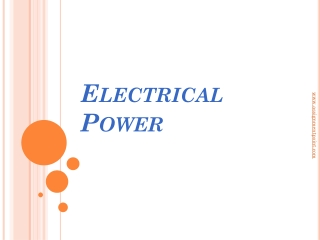DownloadDownload PresentationElectrical Power

# Electrical Power

Télécharger la présentation## Electrical Power

- - - - - - - - - - - - - - - - - - - - - - - - - - - E N D - - - - - - - - - - - - - - - - - - - - - - - - - - -
##### Presentation Transcript

1. Electrical Power www.assignmentpoint.com

2. Electrical power Electrical power • Instantaneous electrical power • The instantaneous electrical power P delivered to a component is given by P(t) = V(t).I(t) where P(t) is the instantaneous power, measured in watts(joulesper second) V(t) is the potential difference(or voltage drop) across the component, measured in volts I(t)is the currentthrough it, measured in amperes If the component is aresistor, then: P = V.I = I2 .R where R is the resistance, measured in ohms. www.assignmentpoint.com

3. Average electrical power for sinusoidal voltages • The average power consumed by a sinusoidally-driven linear two-terminal electrical device is a function of the root mean square(rms) values of the voltageacross the terminals and the current through the device, and of the phase angle between the voltage and current sinusoids. That is, P = V.I cosø www.assignmentpoint.com

4. Contd.. Average electrical power for sinusoidal voltages where • P is the average power, measured in watts • I is the root mean square value of the sinusoidal alternating current (AC), measured inamperes • V is the root mean square value of the sinusoidal alternating voltage, measured in volts • φ is the phase anglebetween the voltage and the current sine functions. www.assignmentpoint.com

5. Average electrical power for AC • Average electrical power for AC P • Where v(t) and i(t) are, respectively, the instantaneous voltage and current as functions of time. • For purely resistive devices, the average power is equal to the product of the rms voltage and rms current, even if the waveforms are not sinusoidal. The formula works for any waveform, periodic or otherwise, that has a mean square; that is why the rms formulation is so useful. www.assignmentpoint.com

6. Power in optics Optical power • Inoptics,orradiometry, the term power sometimes refers to radiant flux, the average rate of energy transport by electromagnetic radiation, measured in watts. • The term "power" is also, however, used to express the ability of a lensor other optical device to focuslight. It is measured in dioptres(inverse metres), and equals the inverse of the focal lengthof the optical device. P = ( ‘f ‘ in metres) www.assignmentpoint.com

7. Power of wave • The total power in one wave length of the wave is given by where = mass per unit length, = wave length, A = amplitude. As the wave moves along the string, this amount of energy passes by a given point on the string during one period of the oscillation. www.assignmentpoint.com

8. Contd.. Power of wave • Thus the power (or) rate of energy transfer associated with the wave is Thus, P v ; P ; P A2. www.assignmentpoint.com